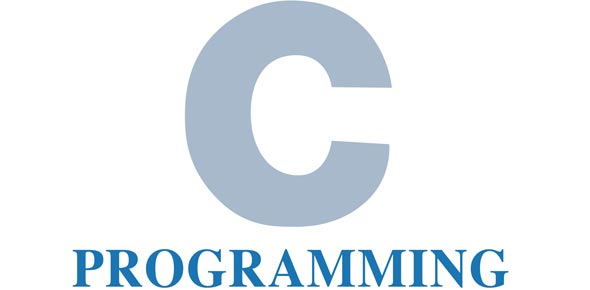# C++ Programming Quiz 1

18 Questions | Total Attempts: 263SettingsC++ is a general purpose programming language with imperative, object oriented and generic programming features. If you just started on this language and wish to get some practice material to test your understanding, take up the quick C++ programming quiz 1 below and keep an eye out for more. Good luck!

• 1.
Who was the programmer for Charles Babbage’s analytical engine?
• A.

Grace Hopper

• B.

• C.

Lord Bryan

• D.

Walter Savitch

• 2.
An algorithm is
• A.

The inputs and outputs of a program

• B.

The part of the computer that does the processing

• C.

A finite set of steps to solve a problem

• D.

A complete computer program

• 3.
• A.

Where a variable is stored

• B.

Where the computer is located

• C.

A step in the program.

• D.

Where the CPU is stored.

• 4.
Which of the following is not part of the Software Life Cycle?
• A.

Analysis

• B.

Design

• C.

Data Entry

• D.

Implementation

• E.

Testing

• 5.
What does the following line of code display to the screen? cout <<  “This is the computer\n programming book\n”;
• A.

This is the computer programming book

• B.

This is the computer

• C.

Nothing

• D.

This is the computer programming book

• 6.
C++ statements end with a
• A.

Period

• B.

Comma

• C.

Semicolon

• D.

Nothing

• 7.
Which of the following is not an example of a program bug?
• A.

Run-time error

• B.

Operator error

• C.

Syntax error

• D.

Logic error

• 8.
The output of the compiler is called
• A.

The program

• B.

Source code

• C.

• D.

Object code

• 9.
A finite sequence of  precise instructions that leads to a problem solution is
• A.

A program

• B.

The CPU

• C.

A List of Inputs and Outputs

• D.

An Algorithm

• 10.
Int number;
• A.

Is an output statement

• B.

Is an input statement

• C.

Is a variable declaration

• D.

Is a program

• 11.
Cout << “How many items would you want?\n”;
• A.

Is an output statement

• B.

Is an input statement

• C.

Is a variable declaration

• D.

Is a program

• 12.
Cin >> number;
• A.

Is an output statement

• B.

Is an input statement

• C.

Is a variable declaration

• D.

Is a program

• 13.
#include <iostream>
• A.

Is a variable declaration

• B.

An executable statement

• C.

An include directive

• D.

Illegal code

• 14.
What is wrong with the following statement? cout  << “Hello to everyone\n;
• A.

Cout should be count

• B.

Missing a semicolon

• C.

Missing a “

• D.

Missing a (

• 15.
C++ is an example of a
• A.

Machine language

• B.

High-level language

• C.

Low-level language

• D.

Assembly language

Related TopicsBack to top